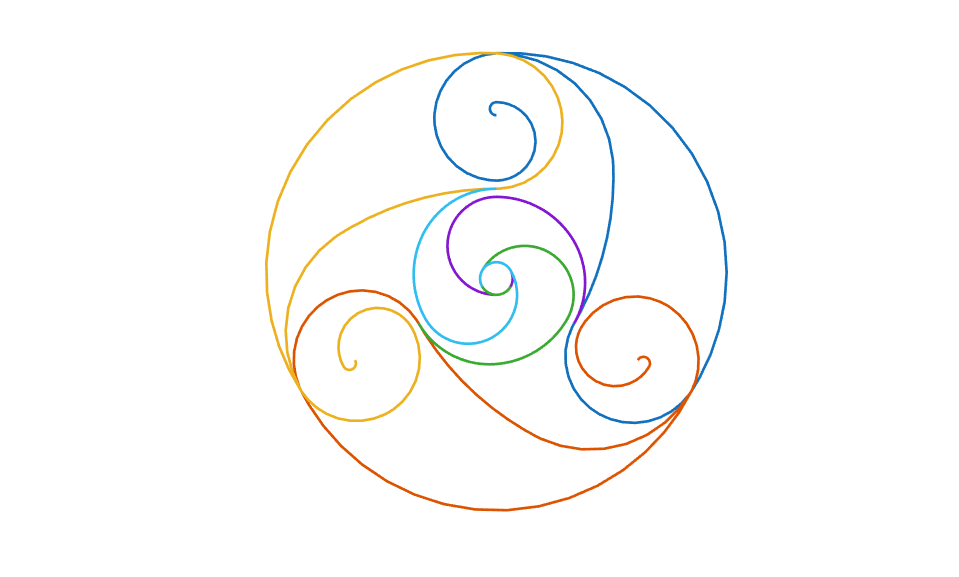Documentation

## Splines in the Plane

This example shows how to use the `spmak`, `spcrv`, `cscvn` and `rscvn` commands from Curve Fitting Toolbox™ to construct spline curves in the plane. This includes plotting tangents and computing the area enclosed by a curve.

### A Simple Spline Curve

Curve Fitting Toolbox can handle vector-valued splines. A d-vector-valued univariate spline provides a curve in d-space. In this mode, `d = 2` is most common, as it gives plane curves.

Here is an example, in which a spline with 2-dimensional coefficients is constructed and plotted.

```knots = [1,1:9,9]; curve = spmak( knots, repmat([ 0 0; 1 0; 1 1; 0 1 ], 2,1).' ); t = linspace(2,8,121); values = fnval(curve,t); plot(values(1,:),values(2,:),'LineWidth',2); axis([-.4 1.4 -.2 1.2]), axis equal title('A Spline Curve'); hold on ```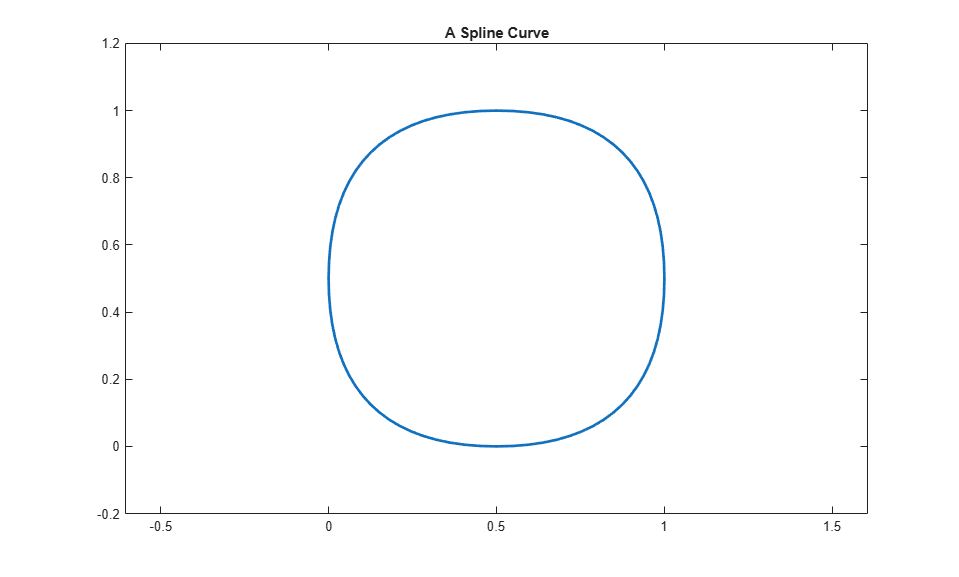### A Word of Caution

You may have noticed that this example did not use `fnplt` to plot the curve, but instead plotted some points on the curve obtained by `fnval`. Here is the code again:

``` t = linspace(2,8,121); values = fnval(curve,t); plot(values(1,:),values(2,:),'LineWidth',2)```

Using `fnplt` directly with this particular spline curve gives the red curve in the figure below.

```fnplt(curve,'r',.5); title('The Full Spline Curve, in Red') ```The explanation?

The spline is of order 4, yet the end knots in the knot sequence

```knots ```
```knots = 1 1 2 3 4 5 6 7 8 9 9 ```

only have multiplicity 2. Therefore, all the B-splines of order 4 for this knot sequence are 0 at the endpoints of the basic interval. This makes the curve start and stop at (0,0).

### A Remedy

Since, in this case, we are really interested only in the curve segment corresponding to the parameter interval [3 .. 7], we can use `fnbrk` to extract that part, and then have no difficulty plotting it, in yellow, with `fnplt`.

```mycv = fnbrk(curve,[3 7]); fnplt(mycv,'y',2.5); title('The Spline Curve of Interest, in Yellow') ```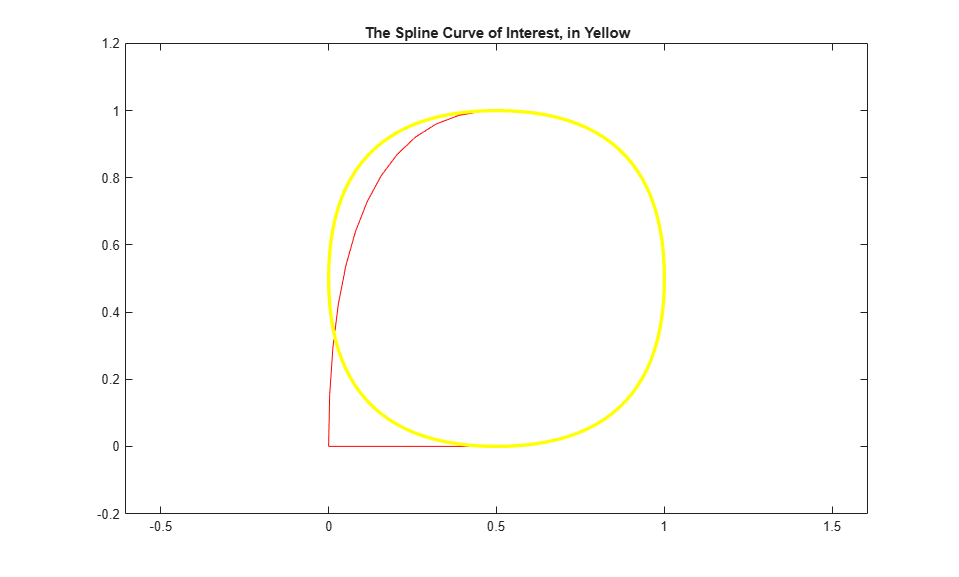### The Area Enclosed By This Curve

Since you now have a spline, namely `mycv`, that describes the curve (and nothing else), you can easily compute the area enclosed by this closed curve, as follows.

```area = diff(fnval(fnint( ... fncmb(fncmb(mycv,[0 1]),'*',fnder(fncmb(mycv,[1 0]))) ... ),fnbrk(mycv,'interval'))) ```
```area = -0.8333 ```

With a little effort, you can recognize this as the value of the integral

` int y(t) d(x(t)) = int y(t) Dx(t) dt`

over the basic interval of the spline `mycv`, with `(x(t),y(t)) := fnval(mycv,t)` the point on the curve corresponding to the parameter value `t`. Here, `fncmb(mycv,[1,0])`, `fncmb(mycv,[0,1])` describe the two components of the spline curve, i.e., the scalar-valued splines `x` and `y`.

Also, the curve is roughly a circle with radius 1/2. Hence, you would expect an area of, roughly,

```disp(pi/4) ```
``` 0.7854 ```

But why is the computed area negative? Because the area enclosed by the curve lies to the left as one travels on the curve with increasing `t`. To verify this, we draw some tangent vectors.

We redraw the curve and also draw the tangent vector to the curve at some points.

```hold off fnplt(mycv,'y',2.5); hold on t = 3:.4:6.2; cv = fnval(curve, t); cdv = fnval(fnder(curve), t); quiver(cv(1,:),cv(2,:), cdv(1,:),cdv(2,:)); title('A Spline Curve With Some Tangents') axis([-.4 1.4 -.2 1.2]), axis equal ```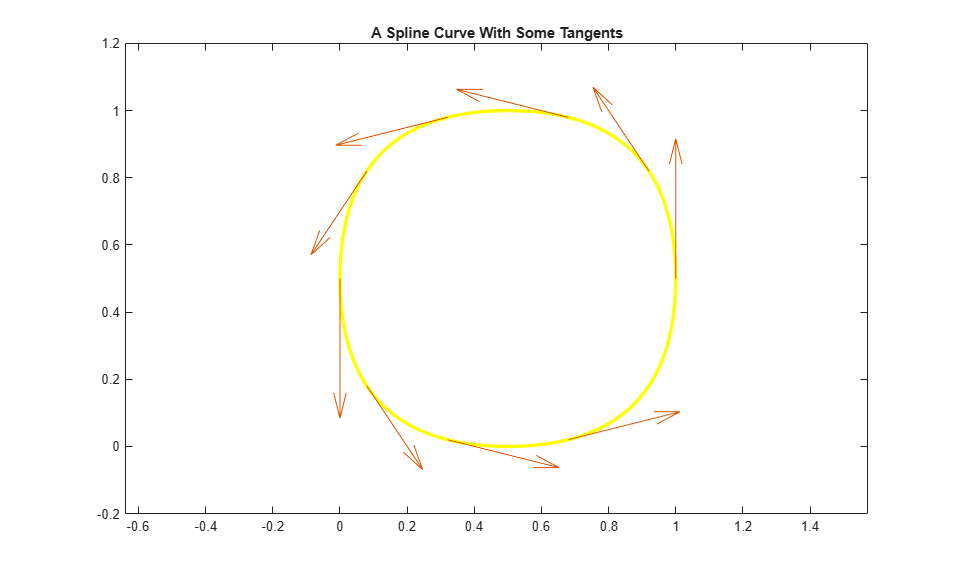### The Intersection of the Curve With a Straight Line

If you wanted to determine the points of intersection of this spline curve with the straight line `y = x`, the following code would give them to you, and plot the segment of that straight line inside the curve:

```cuts = fnval(mycv, ... mean(fnzeros(fncmb(fncmb(mycv,[0,1]),'-',fncmb(mycv,[1,0]))))); plot(cuts(1,:), cuts(2,:),'y','LineWidth',2.5) hold off title('A Spline Curve With Some Tangents and a Cut Across') ```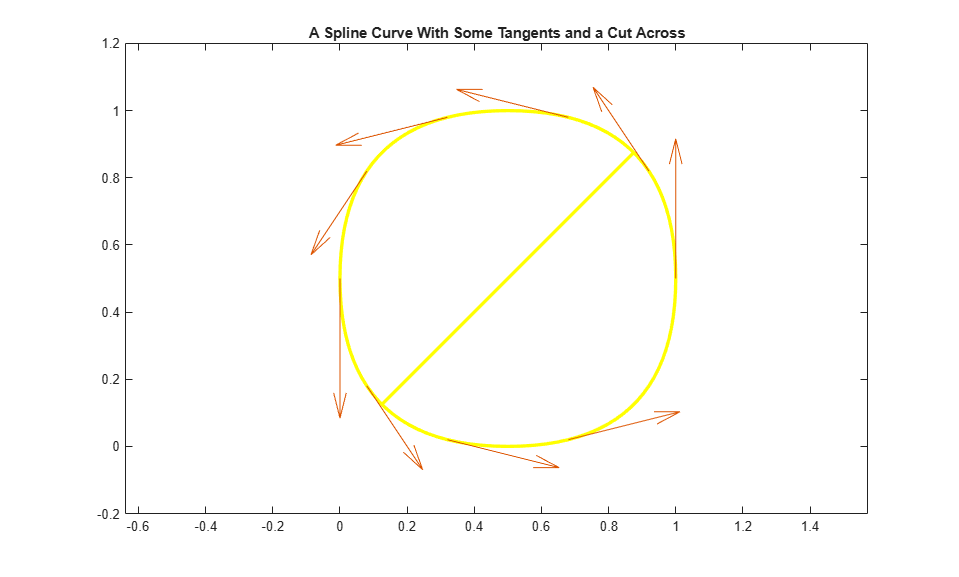### SPCRV: The Control Polygon and the Corresponding Spline Curve

Spline curves are used extensively in the generation of illustrations in which nothing more than a smooth curve of a certain roughly imagined shape is required. For this, Curve Fitting Toolbox contains a special command, `spcrv`, which can be used independently of the rest of the toolbox.

Given a sequence of points in the plane and, optionally, an order `k`, `spcrv` generates, by repeated midpoint knot insertion, the spline curve of order `k` whose control polygon is specified by the given sequence.

The figure below shows such a control polygon, and the corresponding spline curve of order 3.

```points = [0 0; 1 0; 1 1; 0 2; -1 1; -1 0; 0 -1; 0 -2].'; values = spcrv(points,3); plot(points(1,:),points(2,:),'k'); axis([-2 2.25 -2.1 2.2]); hold on plot(values(1,:),values(2,:),'r','LineWidth',1.5); legend({'Control Polygon' 'Quadratic Spline Curve'}, 'location','SE'); ```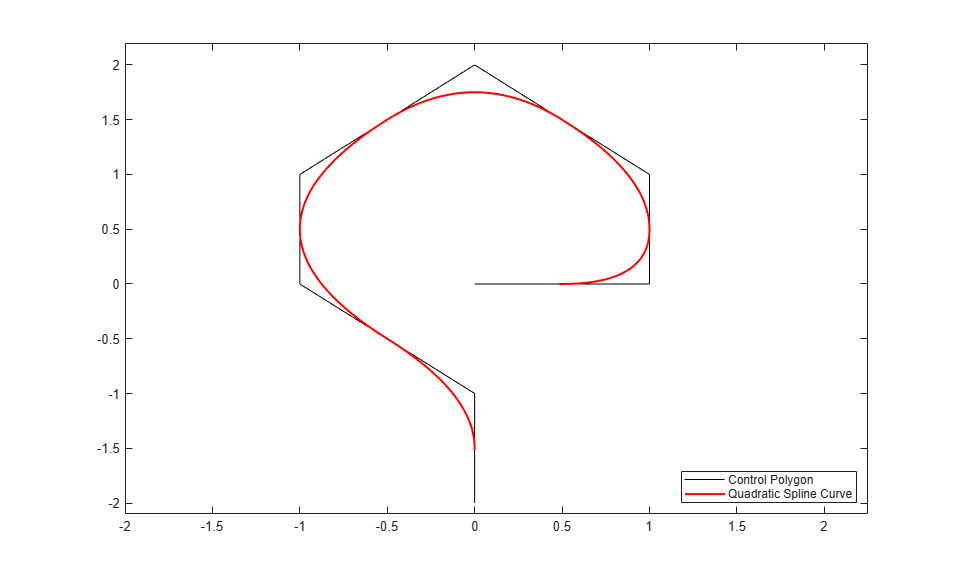Notice that the curve touches each segment of the control polygon at its midpoint, and follows the shape outlined by the control polygon.

### Raising the Order

Raising the order `k` will pull the curve away from the control polygon and make it smoother, but also shorter. Here, we have added the corresponding spline curve of order 4.

```value4 = spcrv(points,4); plot(value4(1,:),value4(2,:),'b','LineWidth',2); legend({'Control Polygon' 'Quadratic Spline Curve' ... 'Cubic Spline Curve'}, 'location','SE'); ```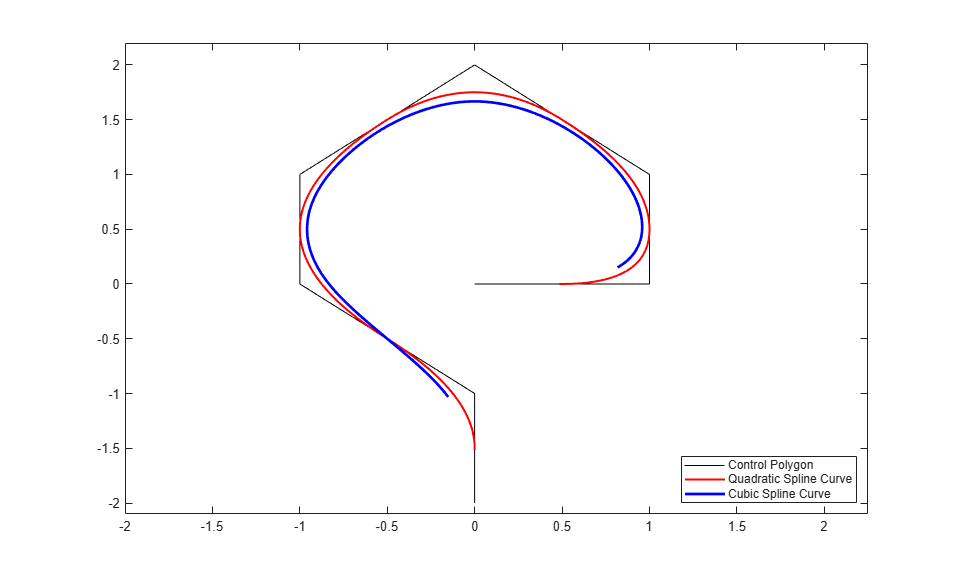### CSCVN

On the other hand, to obtain an interpolating curve, you could use the `cscvn` command, which provides a parametric `natural' cubic spline curve.

```fnplt(cscvn(points), 'g',1.5); legend({'Control Polygon' 'Quadratic Spline Curve' ... 'Cubic Spline Curve' 'Interpolating Spline Curve'}, ... 'location','SE'); ```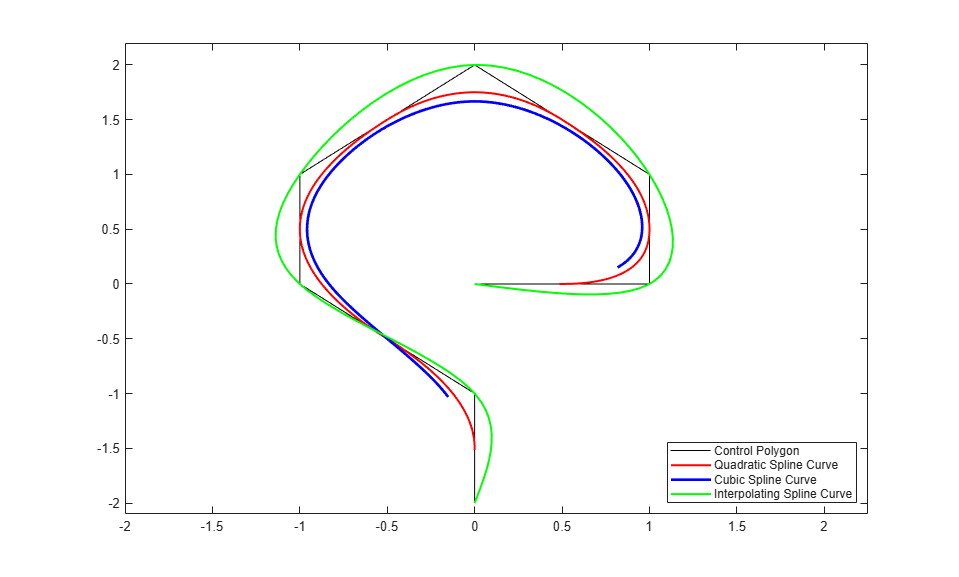By adding the point (.95,-.05) near the second control point, (1,0), we can create an interpolating spline curve that turns faster there.

```np = size(points, 2); fnplt( cscvn([ points(:,1) [.95; -.05] points(:,2:np) ]), 'm',1.5); plot(.95,-.05,'*'); legend({'Control Polygon' 'Quadratic Spline Curve' ... 'Cubic Spline Curve' 'Interpolating Spline Curve' ... 'Faster Turning Near (1,0)'}, ... 'location','SE'); hold off ```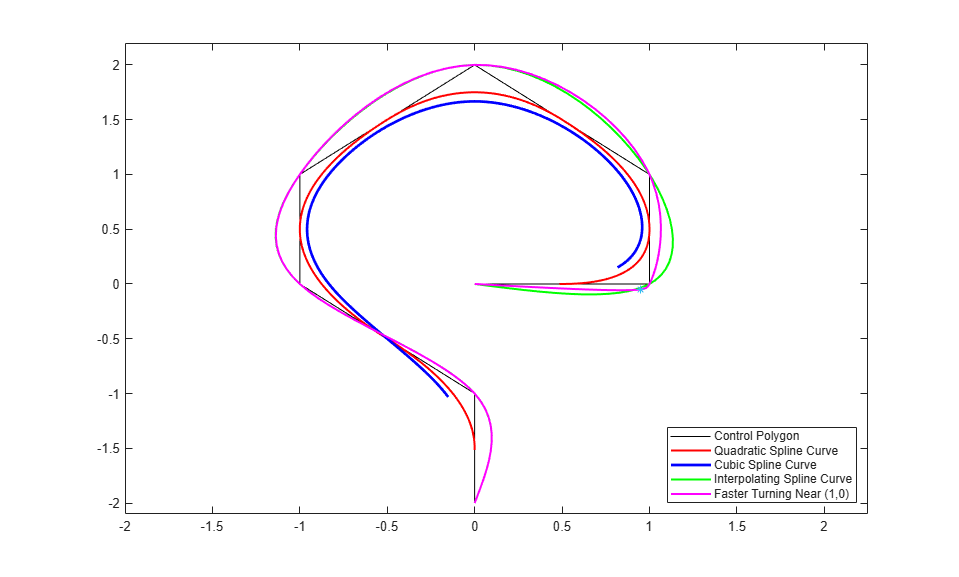### RSCVN

You can also obtain a tangent-continuous curve composed of circular arcs that passes through a given sequence of points in the plane and, optionally, is orthogonal to given normal directions at the points. The command `rscvn` provides such a curve.

For example, the following generates a circle

```c = rscvn([-1 1 -1;0 0 0],[1 1;0 0]); ```

as its plot shows.

```fnplt(c); axis([-1.05 1.05 -1.05 1.05]), axis equal, axis off ```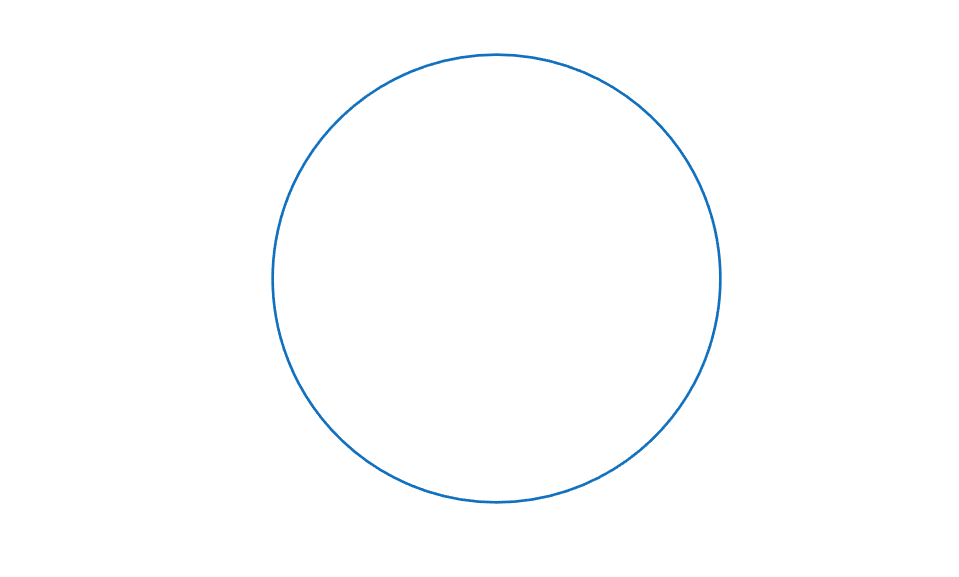`c` is a quadratic rational spline consisting of just two pieces, as the following commands make clear.

```[form, order, breaks] = fnbrk(c,'f','o','b') ```
```form = 'rBform' order = 3 breaks = 0 2 4 ```

It is easy to generate striking patterns with this tool using just a few data points. For example, here is a version of the design on the Bronze Triskele Medallion in the Ulster Museum in Belfast, supposedly done by pieces of circular arcs a long time ago.

```pp =[zeros(1,7); 5.4, 3, 6.9, 2.75, 2.5, .5, 5]; alpha = 2*pi/3; ca = cos(alpha); sa = sin(alpha); c = [ca sa;-sa ca]; d = [0 0 .05 -.05;1 -1 .98 .98]; d = [d c*d]; yin = rscvn([pp(:,[7,1:3]),c*pp(:,3:4),pp(:,3)], d(:,[1 2 1 4 7 5 1])); fnplt(yin), hold on, fnplt(fncmb(yin,c)), fnplt(fncmb(yin,c')) yang = rscvn([pp(:,6),-pp(:,6),pp(:,5),c*pp(:,4)],[d(:,[2 1 1]),c(:,2)]); fnplt(yang), fnplt(fncmb(yang,c)), fnplt(fncmb(yang,c')) axis([-7.2 7.2 -7.2 7.2]), axis equal, axis off, hold off ```